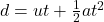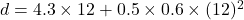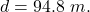(Assume there is no friction) A bike starts rolling down a hill at 4.3 m/s . After 12 s it reaches the bottom moving at 11.5 m/s. How long

Question

(Assume there is no friction) A bike starts rolling down a hill at 4.3 m/s . After 12 s it reaches the bottom moving at 11.5 m/s. How long was the hill? Express your answer with the correct number of significant digits.

in progress 0
5 months 2021-08-22T07:25:15+00:00 1 Answers 8 views 0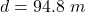Explanation:

Given data:

Initial velocity,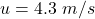Final velocity,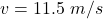Time,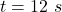The acceleration of the bike can be expressed as,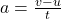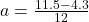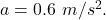The distance of the hill can be given as,